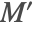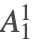# The Wigner-Eckart Theorem

The Wigner-Eckart theorem gives the matrix elements of irreducible tensor operators in terms of their reduced matrix elements:Hereis the 3-symbol and (||||) is the reduced matrix element, which is independent of spatial indices (M,, and q).

 WignerEckart[sys,{op,k,q}] the matrix representation of the operator op with rank k and index q for atomic system sys WignerEckart[sys,{op,k}] thematrix components of the operator op with rank k for atomic system sys WignerEckart[s1,{op,k,q},s2] the matrix element of the operator op between atomic states s1 and s2

Matrix representation of operators.

If the reduced matrix element for an operator is predefined, as for example for the electronic-angular-momentum operator J, this definition is used by WignerEckart to produce explicit matrix elements for the operator.

 An atomic system consisting of a J=1 state.
 The J operator is a rank 1 (κ=1) spherical tensor operator. Thus it has 2κ+1=3 components. This gives the matrices for the J1, J0, and J-1 operators:
 operator rank Zero 0 the zero operator Identity 0 the identity operator Energy 0 the internal (unperturbed) energy operator Dipole 1 the E1 electric dipole operator J 1 the angular momentum operator MagneticMoment 1 the magnetic moment operator Polarizability 0 the effective Hamiltonian for scalar polarizability Polarizability 1 the effective Hamiltonian for vector polarizability Polarizability 2 the effective Hamiltonian for tensor polarizability Polarization κ the polarization operator of rank κ

Some predefined operators.

WignerEckart can be used with an undefined operator: the reduced matrix elements are then given symbolically as ReducedME objects.

 Even though theoperator is undefined, its tensor rank and index determine its matrix elements up to a reduced matrix element.
 ReducedME[s1,{op,k},s2] a symbolic reduced matrix element of the rank-k operator op between atomic states s1 and s2

Symbolic reduced matrix element.

We can complete the definition of a tensor operator by giving a definition for its reduced matrix element. For example, consider a scalar, parity non-conserving interaction mixing two opposite parity states, written in terms of a real, parity-violating mixing parameter δ.

 Define the ReducedME for a scalar PNC operator. The parity-violating matrix elements are imaginary. WignerEckart assumes that symbolic parameters are real, so a factor of  must be included explicitly.
 An atomic system consisting of two J=1 states.
 The matrix for the PNC operator for this system.